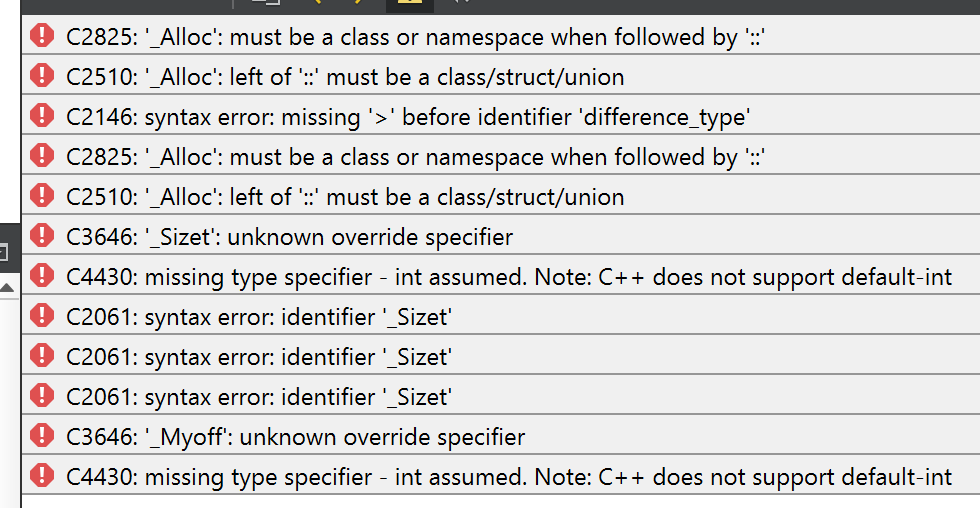# std::swap won't compile?

• on Windows
VS 2017 with MSVC2015 libs
using Creator 4.3.1

i realize this probably isn't a Qt thing, more of a MS thing. here's my code:

``````#include <QCoreApplication>
#include <algorithm>
#include <memory>
#include <utility>

int main(int argc, char *argv[])
{
QCoreApplication	app(argc, argv);

int		a=1, b=2;

std::swap<int>(a, b);

qDebug("a: %d, b: %d\n", a, b);
return app.exec();
}
``````

same code works on mac.
on windows, i get these errors:• OMG is it that easy? where's the "d'oh!" emoji?

• Don't include the template type. See this example.

``````   int a = 5, b = 3;

// before
std::cout << a << ' ' << b << '\n';

std::swap(a,b);

// after
std::cout << a << ' ' << b << '\n';
``````

• And here's the bugreport, it's closed 2013 as By Design :-)

• OMG is it that easy? where's the "d'oh!" emoji?